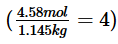Courses

# Revisal Problems For JEE Advanced (Liquid Solutions)

## 32 Questions MCQ Test Chemistry for JEE Advanced | Revisal Problems For JEE Advanced (Liquid Solutions)

Description
This mock test of Revisal Problems For JEE Advanced (Liquid Solutions) for JEE helps you for every JEE entrance exam. This contains 32 Multiple Choice Questions for JEE Revisal Problems For JEE Advanced (Liquid Solutions) (mcq) to study with solutions a complete question bank. The solved questions answers in this Revisal Problems For JEE Advanced (Liquid Solutions) quiz give you a good mix of easy questions and tough questions. JEE students definitely take this Revisal Problems For JEE Advanced (Liquid Solutions) exercise for a better result in the exam. You can find other Revisal Problems For JEE Advanced (Liquid Solutions) extra questions, long questions & short questions for JEE on EduRev as well by searching above.
QUESTION: 1

### Only One Option Correct Type This section contains 17 multiple choice questions. Each question has four choices (a), (b), (c) and (d), out of which ONLY ONE is correct   Q. Mole fraction of non-electrolyte (A) in aqueous solution is 0.07. One molal urea solution freezes at - 1.86°. Thus, depression in freezing point of the non-electrolyte A is

Solution: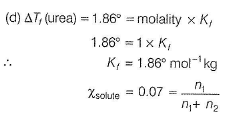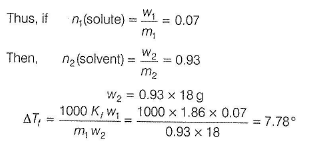QUESTION: 2

### A complex of potassium, iron and cyanide is 100% ionised at 1 m (molal). If the elevation in boiling point is 2.08°, then complex is [Kb (H20 ) = 0.52° mol-1 kg]

Solution: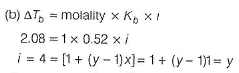where, y = number of ions of the complex

x = degree of ionisation = 1

∴       y = 4

Four ions are formed from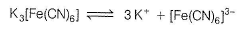QUESTION: 3

### Select the correct statement(s).

Solution: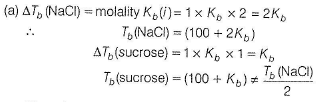Thus, (a) is incorrect

(b) Based on (a),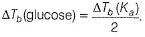Thus, (b) is correct.

(c) Elevation in boiling point (ΔTb) is a colligative property. Boiling point is not a colligative property. Thus, (c) is incorrect.

QUESTION: 4

What mass of sucrose, C12H22011, should you dissolve in 100.0 g water to obtain a solution in which mole fraction of C12H22011 is 0.124?

Solution: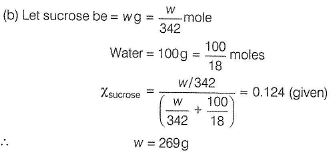QUESTION: 5

The freezing point (in °C) of a solution containing 0.1 g of K3[Fe(CN)6] in 100 g water in which it is 80% ionised is [Kf (H20) = 1.86° mol-1 kg]

Solution: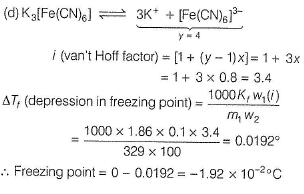QUESTION: 6

Which represents correct difference?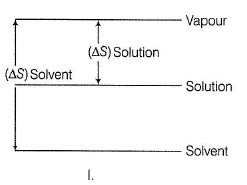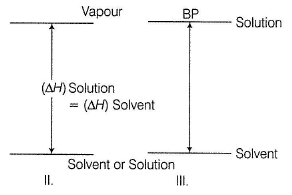Solution:

. (a) (I) Entropy change between solution and vapour is smaller than the entropy change between pure solvent and vapour, thus correct.

(II) Heats of vaporisation for a pure solvent and for a solution are similar because similar intermolecular forces between solvent molecules must be overcome in both cases, thus correct.

(III) When a non-volatile solute is added to a solvent, vapour pressure falls hence, boiling point is elevated, thus correct

QUESTION: 7

60 g of urea is dissolved in 1100 g of solution in water. To keep ΔTf/Kf as 1 mol kg-1, water separated in the form of ice is

Solution:

(a)

Solute (urea) = 60 g

Solution (urea + water) = 1100 g

∴ Solvent (water) = 1100- 60 = 1040 g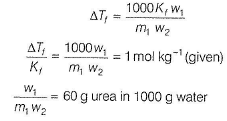Thus, water separated in the form of ice = 1040 - 1000 = 40g

QUESTION: 8

A solution contains 0.1 mole of acetamide in 1 L of glacial acetic acid. When the solution is cooled, the first crystal that appeared at the freezing point contains the molecule(s) of

Solution:

(b) When a solute is added to a solvent, freezing point is lowered. Thus, freezing point of solution is less than that of the solvent. Let freezing point of solvent = T1 and freezing point of solution = T2.,

T2 <T1

On lowering temperature, first is attained hence, acetic acid is converted into solid (crystal).

QUESTION: 9

Two moles of the complex (Cu(NH3)3Ci]Cl are dissolved in 3 moles of H20 . Relative decrease in vapour pressure was found to be 0.50. If this solution is treated with aq. AgN03 solution, then there is formation of

Solution: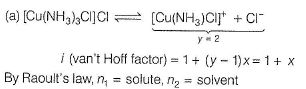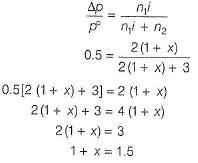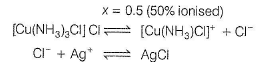Cl- from 2 moles complex = 2 x 0.5 = 1.0

∴ AgCI formed = 1 mol

QUESTION: 10

Total volume of a mixture of 46 g ethanol (C2H5OH) and 72 g water (H20 ) based on the given figure is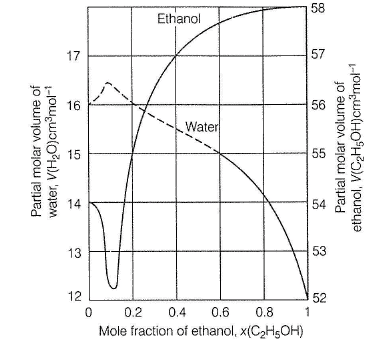Solution: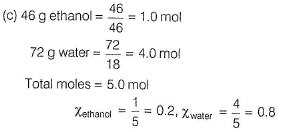From the graph, partial molar volume of ethanol when

(X = 0.2) = 55cmmol-1

and that of water when ( x = 0.8) = 14cm3mol-1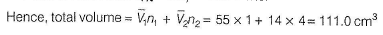QUESTION: 11

pH of 0.1 M dibasic acid is found to be 3. Hence, its osmotic pressure at temperature T K is

Solution: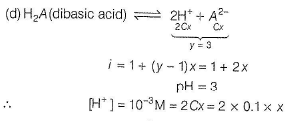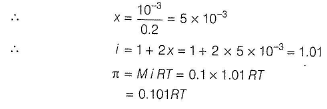QUESTION: 12

Water is purified by ion-exchange resin (RH2) in which Ca2+ in hard water is replaced by H+

RH2 + Ca2+ ----- >RCa + 2H+

Water coming out of ion-exchange has pH2. Hence, hardness in ppm of Ca2+ ion is

Solution: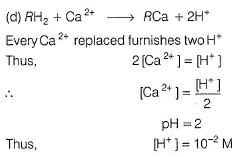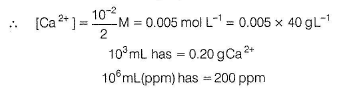QUESTION: 13

A sample of drinking water is contaminated with CHCI3 to the level of 15 ppm by mass. Thus, mass percentage and molality, respectively are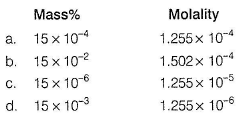Solution: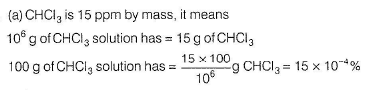106g of CHCI3 solution has = 15 g of CHCI3 (106 - 15)g H2O = 106g H20 has = 15 g of CHCI3

103 g of H2O has = 15 x 10-3g CHCI3 =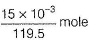∴ Molality = 1.255 x 10-4 mol kg-1

QUESTION: 14

Based on the given dia gram , which of the fo llow ing statem ents regarding the solu tions of two miscible volatile liquids A and B are correct?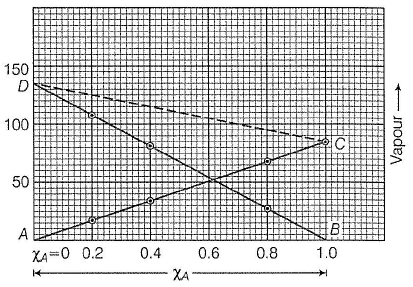I. Plots AC and BD show that Raoult’s law is obeyed for the solution in which A is solute and B is solvent and as well as for that in which B is solute and A is solvent.

II. Plots CD shows that Dalton’s law of partial pressure is observed for the binary solution of components A and 6.

III. In pure state, vapour pressures of volatile liquid differ by 50 units.

IV. Vapour pressure of a mixture containing 40 mole % of B is about 105 units.

Select the correct answer using the codes given below.

Solution: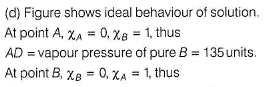BC = vapour pressure of pure A = 85 units

As A is added into 6, vapour pressure of A decreases and that of 6 increases.

Thus, AC shows Raoult's law for a solution of S in A (solvent) and SO shows Raoult's law for a solution of A in B (solvent).

Thus, (I) is correct.

Total vapour pressure at any composition is given by line CD, thus (II) is correct.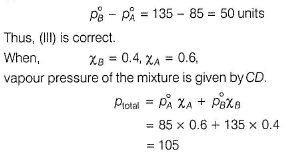it is same as given by graph.
Thus, (IV) is also correct.

QUESTION: 15

25 mL of an aqueous solu tion of KCI was fo und to require 20 mL of 1.0 M AgN03 solution when titrated using K2Cr04 as an indicator. Thus, d epression in freezing point of KCI solution with 100% ionisation will be

[Kf (H20 ) = 2.0° mol-1 kg, molarity = molality]

Solution:

(b)

Ag + + Cl- → AgCI

When titration is complete,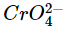gives chocolate coloured precipitate with Ag+.

2Ag+ +→ Ag2Cr04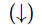This end point is detected.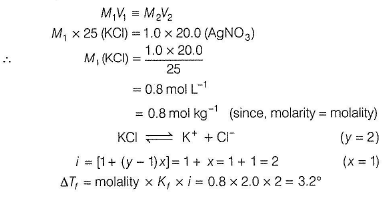QUESTION: 16

A 0.001 molal solution of [Pt(NH3)4CI4] in water had a depression in freezing point of 0.0054°. Kf (H20 ) = 1.86° mol-1 kg (assume 100% ionisation). Thus, com plex is

Solution:

(c)

Let total number of ions due to ionisa tion of the complex = y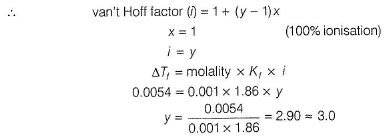NH3 is always a ligand, hence Cl can be ligand or ionisable ions.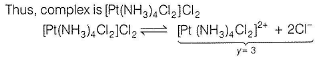QUESTION: 17

The complete combustion of 2.40 g of a compound of carbon, hydrogen and oxygen yielded 5.46 g of C02 and 2.23 g of H20 . When 8.69 g o f the compound was dissolved in 281 g of water, the freezing point depression was found to be 0.97°C. Thus, molecular formula of the organic compound is [Kf (H20 ) = 1.86° mol-1 kg)]

Solution: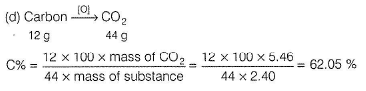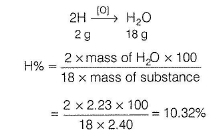Oxygen (by difference) = 100 -(62.05 + 10.32)= 27.63%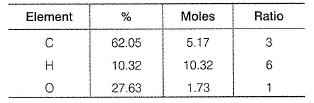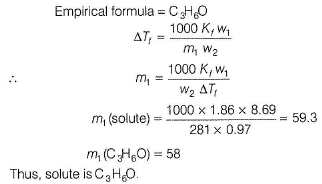*Multiple options can be correct
QUESTION: 18

One or More than One Options Correct Type
This section contains 4 multiple type questions. Each question has 4 choices (a), (b), (c) and (d), out of which ONE or MORE THAN ONE are correct.

Q.

You have been provided with 10.00% by mass solution of ethanol in water.

At 15°C, density = 0.9831 g mL-1

At 25°C, density = 0.9804 g mL-1

Select the correct statement(s).

Solution:

(a, c)

10% ethanol by mass means

100 g (ethanol + water) solution has = 10 g ethanol

90 g H2O has ethanol = 10 g ethanol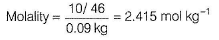Molality does not involve density, hence same at 15°C and 25°C.
Thus, (c) is correct.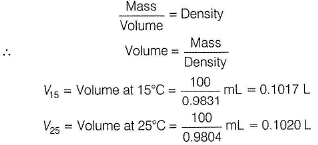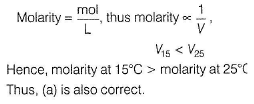*Multiple options can be correct
QUESTION: 19

One molal aqueous glucose solution has density 1 .180 gm L-1. Also, Kf / Kb = 3 . Thus,

Solution:

Glucose solution is = 1 mol kg-1 water

Solute (glucose) = 180g

Solvent (water) = 1000 g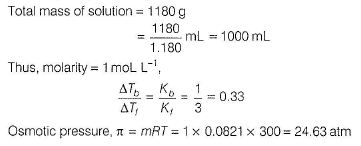*Multiple options can be correct
QUESTION: 20

For a mixture of binary c om ponents A and 8 with vapo ur pressures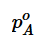and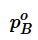in pure state, total vapour pressure is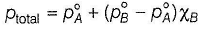where, XB is mole fraction of component B in the mixture. From this equation, it is concluded that

Solution: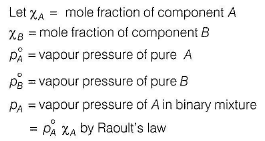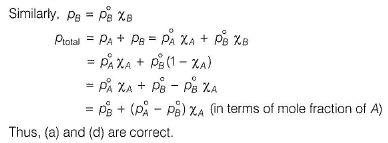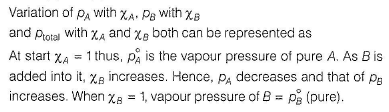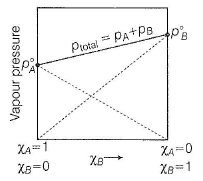Thus, vapour pressure of 6 increase as Xs increases and decrease as %A decreases.
Line joining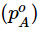and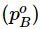represent total vapour pressure.
Thus, (b) and (c) are also correct.
Note Vapour pressure of Ais less than vapour pressure of B. Hence, A is less volatile than B. (d) pt0(a| can also be expressed as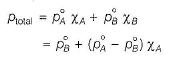*Multiple options can be correct
QUESTION: 21

If liquids A and B form an ideal solution, then

Solution:

(a, c)

Two liquids (A, B) form an ideal solution, when force of attraction between A and 6 (in mixture) is same as between A and A and between 6 and 6. In such case,  Δ Hmix= 0.
Since, disorder increases,  Δ Smix. Also, process is spontaneous at a given temperature,

ΔG = ΔH - T ΔS = 0 — T ΔS
ΔGmix < 0

QUESTION: 22

Comprehension Type
This section contains 3 paragraphs, each describing theory, experiments, data, etc. Two questions related to the paragraph have been given.six question related to paragraphs have been given Each question has only one correct answer out of the given 4 options (a), (b), (c) and (d)

Passage 1

Water boils at 100°C at 1 atm pressure. Latent heat of vaporisation (ΔH ) = 10 kcal mol-1.

Q.

What is the vapour pressure at 40°C?

Solution:

Variation of vapour pressure with temperature is given Clapeyron-Clausius equation.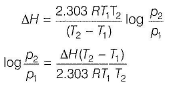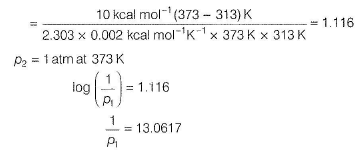p1 = 0.07656 atm

p1 = 0.07656 x 760 torr = 58.19torr

Vapour pressure at 40°C(313 K) = 58.19 torr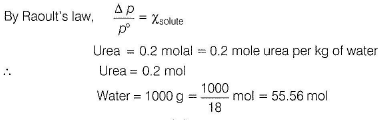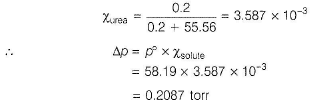QUESTION: 23

Passage l

W ater boils at 100°C at 1 atm pressure. Latent heat of vaporisation (ΔH ) = 10 kcal mol-1.

Q.

Lowering of vapour pressure of 0.2 molal urea solution at 40°C is

Solution:

Variation of vapour pressure with temperature is given Clapeyron-Clausius equation.p1 = 0.07656 atm

p1 = 0.07656 x 760 torr = 58.19torr

Vapour pressure at 40°C(313 K) = 58.19 torrQUESTION: 24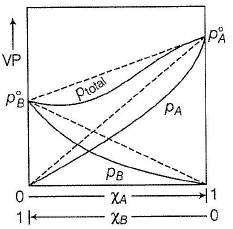Vapour pressure diagram for real solutions of two liquids A and B that exhibit a negative deviation from Raoult’s law. The vapour pressure of both A and 8 are less than predicted by Raoult’s law. The dashed lines represent the plots for ideal solutions.

Q.

This type of deviation is also expected in the following mixture.

Solution: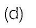This is a case of negative deviation, thus force of attraction between (A ... B) is larger than average of (A ... A) and (B ... B). Thus, vapour pressure of mixture is decreased, boiling point is increased, hence

ΔH < 0, ΔV = negative

Diethylether and chloroform show negative deviation.

QUESTION: 25Vapour pressure diagram for real solutions of two liquids A and B that exhibit a negative deviation from Raoult’s law. The vapour pressure of both A and 8 are less than predicted by Raoult’s law. The dashed lines represent the plots for ideal solutions.

Q.

Solution containing components A and 8 shows this type of deviation from ideal behaviour when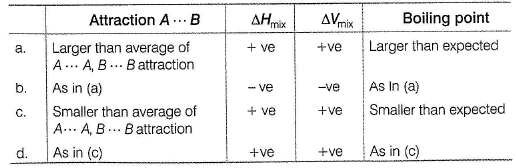Solution: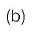This is a case of negative deviation, thus force of attraction between (A ... B) is larger than average of (A .... A) and (B....B).

Thus, vapour pressure of mixture is decreased, boiling point is increased, hence

ΔH < 0, ΔV = negative

Diethylether and chloroform show negative deviation.

QUESTION: 26

Passage III

Vapour pressure of binary solution containing 6 g of non-volatile solute in 180 g water is 20.0 torr at 298 K. If one mole of water is further added, vapour pressure increases by 0.02 torr.

Q.

Molar mass of the solute is

Solution: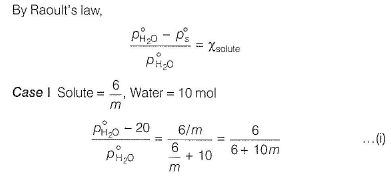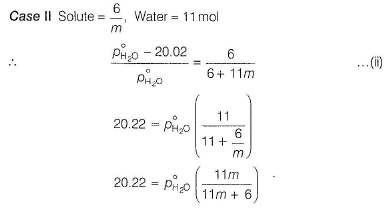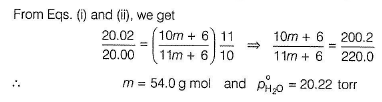QUESTION: 27

Passage III

Vapour pressure of binary solution containing 6 g of non-volatile solute in 180 g water is 20.0 torr at 298 K. If one mole of water is further added, vapour pressure increases by 0.02 torr

Solution:*Answer can only contain numeric values
QUESTION: 28

The so-called ozone layer in the earth's stratosphere has an average total pressure of 10 mm with partial pressure of ozone in the layer 1.2 x 10-5 mm. What is the concentration of ozone (in ppm) assuming that air is a mixture of N2 and 02 is 4 : 1 molar ratio.

Solution: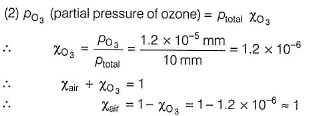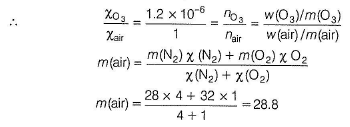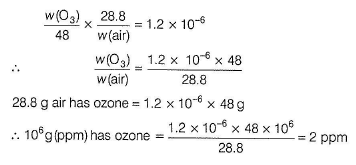*Answer can only contain numeric values
QUESTION: 29

The vapour pressure of a solvent is decreased by 10 mm of Hg when a non-volatile solute was added to the solvent. The mole fraction of the solute in the solution is 0.2. How many times the vapour pressure is decreased when mole fraction of solvent is decreased by 25%?

Solution: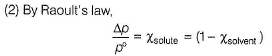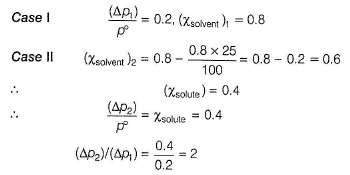*Answer can only contain numeric values
QUESTION: 30

The freezing point depression of a 1.00x 10-3 molal aqueous solution of Kx[Fe(CN)6] is 7.1O x 10-3 K. If Kf (H2O)= 1.86 K kg mol-1, what is the value of x?

Solution: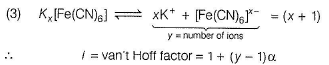∴    i = van't Hoff factor = 1 + ( y - 1 )α

where, α = degree of ionisation = 1 = 1 + (x + 1 — 1) 1 = (x + 1)

ΔTf = molality x Kf x i

7 x 10-3 = 1 x 10-3 x 1.86 x (x + 1)

x + 1 = 3.76 = 4

∴   x = 3

QUESTION: 31

Matching List Type
Choices for the correct combination of elements from Column I and Column II are given as options (a), (b), (c) and (d), out of which one is correct

Q.

17.1 g of sucrose (C12H22011) is dissolved in 1000 g of water.

p°(H20) = 26.50 mm

Kf (H20) = 1.86 mol-1 kg

Kb (H20 ) = 0.52° mol-1 kg

Match the colligative properties in Column I with their values in Column II and select the answers from the codes given below.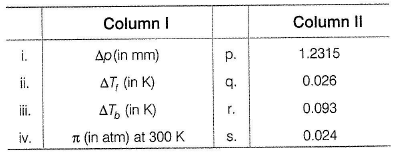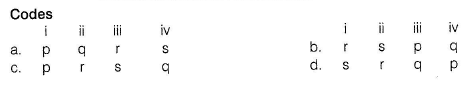Solution: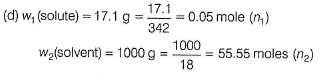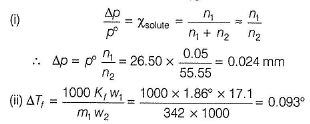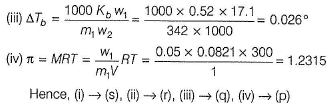*Answer can only contain numeric values
QUESTION: 32

Direction :  This section contains 4 questions. When worked out will result in an integer from 0 to 9 (both inclusive).

Q.

Molarity of Na+ ions in lake water is 4.58 mol L-1.Density of lake water is 1.25 g mL-1.
Thus, molality of Na+ ion i s ...... molal.

Solution:

(4) Molarity = 4.58 mol L-1

1000 mL has 4.58 mol Na + ions
m/V= Density
∴ Mass of solution = V x d = 1000 x 1.25 g = 1250 g

Na+ ions = 4.58 mol = 4.58 x 23 g = 105.34 g

∴H20 in lake water = 1250 - 105.34= 1144.66g= 1.145 kg

∴ Molality =